# Right Triangles and Trigonometry

Lesson 17

Math

Unit 4

Lesson 17 of 19

## Objective

Verify algebraically and find missing measures using the Law of Sines.

## Common Core Standards

### Core Standards

• G.SRT.D.10 — Prove the Laws of Sines and Cosines and use them to solve problems.

• 7.RP.A.2

## Criteria for Success

1. Describe why trigonometric ratios cannot be applied directly in non-right triangles.
2. Describe how auxiliary altitudes are beneficial in establishing additional relationships in non-right triangles.
3. Write the height of the altitude in terms of sine of angles in the non-right triangle.
4. Using the transitive property, establish that the Law of Sines of $${\frac{\mathrm{sin}A}{a}=\frac{\mathrm{sin}b}{b}=\frac{\mathrm{sin}c}{c}}$$ describes the relationships in non-right triangles.
5. Use this relationship to solve triangles.
6. Describe the ambiguous case for the Law of Sines.

## Tips for Teachers

Anchor Problem #2 will require the teacher to do a significant amount of modeling to define and algebraically verify the Law of Sines. Reference EngageNY, Geometry, Module 2, Lesson 32: Teacher Version (Discussion) for more guidance on this.

Fishtank Plus

Unlock features to optimize your prep time, plan engaging lessons, and monitor student progress.

## Anchor Problems

### Problem 1

a. Find the lengths of $$d$$ and $$e$$.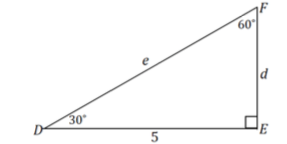b. Why can't you use the same method to find the lengths of $$x$$ and $$y$$?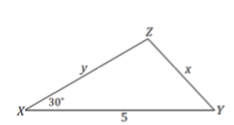#### References

EngageNY Mathematics Geometry > Module 2 > Topic E > Lesson 32Opening Exercise

Geometry > Module 2 > Topic E > Lesson 32 of the New York State Common Core Mathematics Curriculum from EngageNY and Great Minds. © 2015 Great Minds. Licensed by EngageNY of the New York State Education Department under the CC BY-NC-SA 3.0 US license. Accessed Dec. 2, 2016, 5:15 p.m..

Modified by Fishtank Learning, Inc.

### Problem 2

There is a rule, the Law of Sines, which allows you to use trigonometric ratios to determine side lengths and angles of non-right triangles.

Law of Sines:
Given $${\triangle ABC}$$,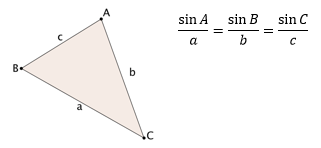Using the two congruent triangles below and the altitudes drawn, show that the Law of Sines is true.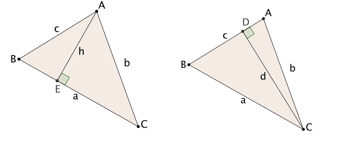#### References

EngageNY Mathematics Geometry > Module 2 > Topic E > Lesson 32Discussion

Geometry > Module 2 > Topic E > Lesson 32 of the New York State Common Core Mathematics Curriculum from EngageNY and Great Minds. © 2015 Great Minds. Licensed by EngageNY of the New York State Education Department under the CC BY-NC-SA 3.0 US license. Accessed Dec. 2, 2016, 5:15 p.m..

### Problem 3

In $${\triangle ABC}$$$${a=9}$$$${c=12}$$, and $${\angle A=30^\circ}$$. Determine the missing angle and side measurements.

## Problem Set

The following resources include problems and activities aligned to the objective of the lesson that can be used to create your own problem set.

• Include problems where students reenact the Law of Sines on their own.
• Be sure to include an example that you highlight during independent practice that deals with the ambiguous case where a unique triangle is not given via the Law of Sines.

Use the Law of Sines to find the lengths $$b$$ and $$c$$ in the triangle below. Round answers to the nearest tenth as necessary.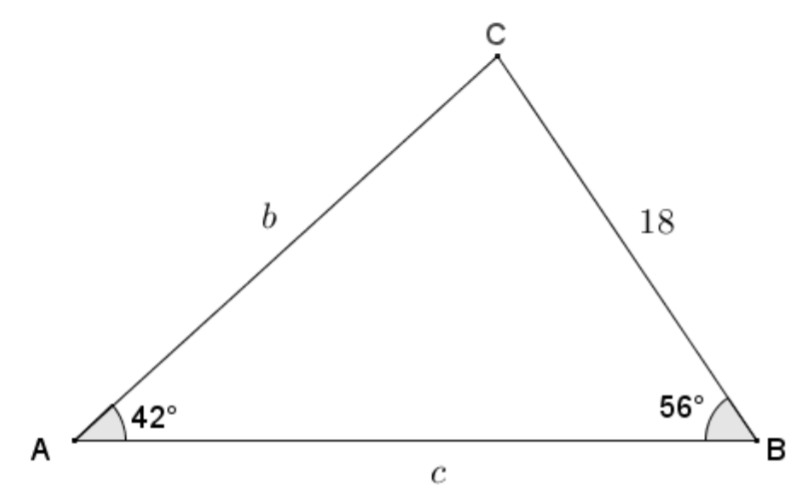#### References

EngageNY Mathematics Geometry > Module 2 > Topic E > Lesson 32Exit Ticket, Question #1

Geometry > Module 2 > Topic E > Lesson 32 of the New York State Common Core Mathematics Curriculum from EngageNY and Great Minds. © 2015 Great Minds. Licensed by EngageNY of the New York State Education Department under the CC BY-NC-SA 3.0 US license. Accessed Dec. 2, 2016, 5:15 p.m..

Lesson 16

Lesson 18

## Lesson Map

Topic A: Right Triangle Properties and Side-Length Relationships

Topic B: Right Triangle Trigonometry

Topic C: Applications of Right Triangle Trigonometry

Topic D: The Unit Circle

Topic E: Trigonometric Ratios in Non-Right Triangles